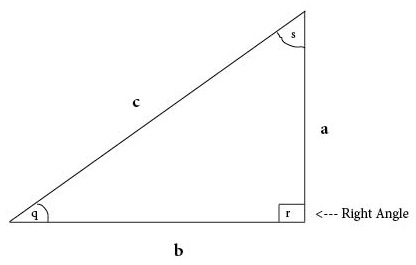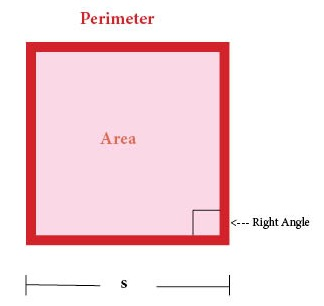# Pythagorean Theorem

### a²  +  b²  =  c²# Rectangle

Rectangle = Flat Shape with Four Sides (with four right angles)

# Cube

Cube = Three-Dimensional Shape with Six Square Faces (with all right angles)# Square

Square = Flat Shape with Four Equal Sides (with four right angles)